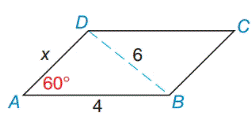Chapter 11.4, Problem 27E### Elementary Geometry for College St...

6th Edition
Daniel C. Alexander + 1 other
ISBN: 9781285195698

#### Solutions

Chapter
Section### Elementary Geometry for College St...

6th Edition
Daniel C. Alexander + 1 other
ISBN: 9781285195698
Textbook Problem
1 views

# In Exercises 23 to 28, use a form of the Law of Cosines to find the measure of the indicated side or angle. Angle measures should be found to the nearest degree and lengths of sides to the nearest tenth of a unit.Parallelogram ABCD

To determine

To find:

The value of x the given figure by using the Law of Cosines.

Explanation

Formula:

The Law of Cosines

cosα=b2+c2a22bc

cosβ=a2+c2b22ac

cosγ=a2+b2c22ab

Where α, β, and γ is the acute angle of the triangle, and a, b, and c is the sides of the triangle.

Calculation:

Given,

From the figure,

a=x

b=4

c=6

By the Law of Cosines,

c2=a2+b22abcosγ

(6)2=a2+(4)22(x)(4)cos60°

36=x2+(4)22(x)(4)

### Still sussing out bartleby?

Check out a sample textbook solution.

See a sample solution

#### The Solution to Your Study Problems

Bartleby provides explanations to thousands of textbook problems written by our experts, many with advanced degrees!

Get Started

#### Find more solutions based on key concepts